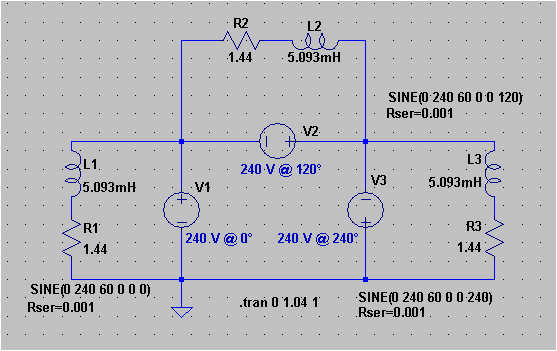# 3-Phase Circuits (Wye/Delta)

• Engineering
JGrecs

## Homework Statement

each phase of a three phase delta connected generator supplies a full load current of 100A at a voltage of 240V and at a power factor of 0.6 lagging.

Find:
a) line voltage
b) line current
c) the kVA rating
d) the KW

2. The attempt at a solution

a) Line voltage = Phase Voltage (in delta)

VP= VL/1.732
VP= 240/1.732
VP= 138.5V

Therefore the line voltage is 138.5 volts.

b) Phase current at full load= 100A

Line current= Phase current x 1.732

IL= (100)(1.732)
IL= 173.2A

Therefore the line current is 173.2 amps.

c) kVA= (1.732)(VL)(IL)/1000

=(1.732)(240)(173.2)/1000
= 700VA

d) P= (1.732)(VL)(IL)(0.6)

= (1.732)(240)(173.2)(0.6)
= 43kW

How do these answers look? I am a little unsure of which voltages/currents to substitue into the kVA and P formulas.
Thanks,

Last edited:

Mentor
I may be a bit rusty at power circuits, but aren't the line voltages equal to the phase voltages for a delta-connected generator?

JGrecs
^ Yes. What I am unsure of is question c and d. I am not exactly sure which values to substitute into kVA= (1.732)(VL)(IL)/1000 and P= (1.732)(VL)(IL)(0.6). I used the line current and line voltage for both. Is this right? (assuming that the line current I calculated is correct to begin with.)

Aussielec

a)240 volts
b)100 amps
c)240*100*1.732=41.6KVA
d)240*100*1.732*0.6 =25KW

The thing here is that the question IMO isn't worded very well so that might be half the problem.

Remember when connected in delta the line and phase voltages are the same, while phase and line currents will be different.

When connected in star line and phase voltage will be different, while line and phase currents will be the same.

Is there an actual answer included with the question?

Last edited:
JGrecs

a)240 volts
b)100 amps
c)240*100*1.732=41.6KVA
d)240*100*1.732*0.6 =25KW

The thing here is that the question IMO isn't worded very well so that might be half the problem.

Remember when connected in delta the line and phase voltages are the same, while phase and line currents will be different.

When connected in star line and phase voltage will be different, while line and phase currents will be the same.

Is there an actual answer included with the question?

No answers provided. You say for b), that the line current is 100A. Does this mean that the Load current=Line current for delta connections? Also for question a), If I am not mistaken, the phase voltage is calculated Vload/1.732 which comes out to 138.5V. So shouldn't VLine be 138.5V?

Last edited:
Aussielec
When connected in delta line and phase current will be different. But this what I can't understand- Where is this 100 amps measured from? The generator windings or the line?

The question reads. "supplies a full load current of 100A"? But from where?

JGrecs
When connected in delta line and phase current will be different. But this what I can't understand- Where is this 100 amps measured from? The generator windings or the line?

The question reads. "supplies a full load current of 100A"? But from where?

each phase of a three phase delta connected generator supplies a full load current of 100A

The Windings?

100A per phase/winding

Last edited:
Mentor
As I said, I'm a bit rusty on multiphase power system measurement/specification conventions. But if we were to assume that the load consists of identical impedances across the phases supplies, and each is drawing 100A with a power factor of 0.6, then we could determine the appropriate impedance (pf corresponds to 53.13° lag angle for the current) and create a Spice model:[note that the ground is for the convenience of the simulation software]

Each load will draw its 100A, and each phase voltage source will produce 100A, all shifted according to the phase angles of the supplies.

In order to answer the given problem, I would first have to recall or find out: does a single impedance and the power it dissipates characterize the system, or should the power phasors of each load be summed to find total kVA and kW numbers?

#### Attachments

JGrecs
Ok,

A) According to textbook: VLoad=VPhase=VLine (Delta)

Therefore VLine in this case = 240V

B) According to textbook: ILine=IPhase X 1.732 (Delta)

IPhase=100A (Would you agree)?

Therefore ILine= 173.2A

c)

kVA= kW/PF

kVA= 43/0.6
kVA= 72kVA

or

kVA= 240 X 100 X 1.732
kVA= 72kVA

or

kVA= 240 X 1.732 X 3
kVA= 72kVA

D) Textbook: P= 1.732 X VLine X ILine X PF or P= 3 X VPhase X IPhase X PF

P= 1.732 X 240V X 173.2A X 0.6
P= 43kW

or

P= 3 X 240V X 100A x 0.6
P= 43kW

These should all be correct. Any objections brehs?

Last edited:
JGrecs
Thanks for the help, Gneill & Aussielec.

Mentor
Your results look okay to me!